# Forced Convective Heat Transfer (Part - 3) Chemical Engineering Notes | EduRev

## Chemical Engineering : Forced Convective Heat Transfer (Part - 3) Chemical Engineering Notes | EduRev

The document Forced Convective Heat Transfer (Part - 3) Chemical Engineering Notes | EduRev is a part of the Chemical Engineering Course Heat Transfer for Engg..
All you need of Chemical Engineering at this link: Chemical Engineering

Illustration 4.1
Pressurized air is to be heated by flowing into a pipe of 2.54 cm diameter. The air at 200oC and 2 atm pressure enters in the pipe at 10 m/s. The temperature of the entire pipe is maintained at 220oC. Evaluate the heat transfer coefficient for a unit length of a tube considering the constant heat flux conditions are maintained at the pipe wall. What will be the bulk temperature of the air at the end of 3 m length of the tube?

The following data for the entering air (at 200oC) has been given,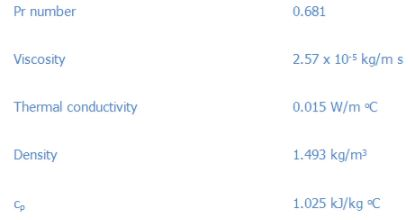Solution 4.1

Reynolds number can be calculated from the above data,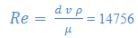The value of Reynolds number shows that the flow is in turbulent zone. Thus the Dittus-Boelter equation (eq.4.3) should be used,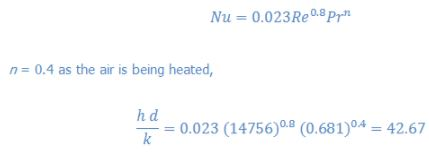Thus h can be calculated for the known values of k, and d, which comes out to be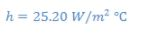Energy balance is required to evaluate the increase in bulk temperature in a 3 m length of the tube,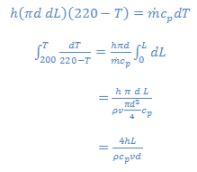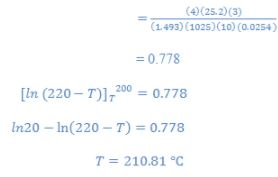Therefore the temperature of the air leaving the pipe will be at 210.81oC.

4.3.2 Laminar flow
Hausen presents the following empirical relations for fully developed laminar flow in tubes at constant wall temperature.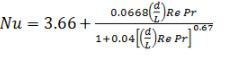(4.8)

The heat transfer coefficient calculated from eq. 4.8 is the average value over the entire length (including entrance length) of tube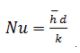.
Sieder and Tate suggested a simple relation for laminar heat transfer in tubes.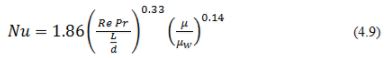The condition for applicability of eq. 4.9: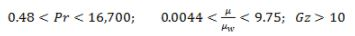where, μ is the viscosity of the fluid at the bulk temperature and μw is that at the wall temperature Tw . The other fluid properties are at mean bulk temperature of the fluid. Here also the heat transfer coefficient calculated from eq. 4.9 is the average value over the entire length (including entrance length) of tube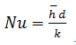.

The empirical relations shown in eq. 4.2-4.9 are for smooth pipe. However, it case of rough pipes, it is sometimes appropriate that the Reynolds analogy between fluid friction and heat transfer be used to effect a solution under these conditions and can be expressed in terms of Stanton number.

In order to account the variation of the thermal properties of different fluids the following equations may be used (i.e. Stanton number multiplied by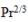),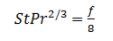(4.10)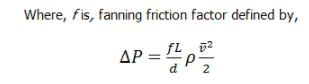(4.11)

where,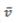is the mean free velocity. The friction factor can be evaluated from Moody’s chart.

4.3.3 Flow through non-circular ducts
The same co-relations as discussed in section 4.4.1 can be used for the non-circular ducts. However, the diameter of the tube has to be replaced by the hydraulic diameter or equivalent diameter for the non-circular ducts. The hydraulic diameter is defined as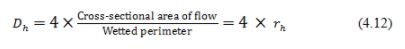4.3.4. Flow over a flat plate
Heat transfer in flow over a plate occurs through the boundary layer formed on the plane. Therefore at any location the heat transfer coefficient will depend on the local Reynolds and Prandtl number. For local heat transfer coefficient in laminar boundary layer flow, the following correlation can be used to find the local Nusselt number. It depends upon the distance from the leading edge (x) of the plate.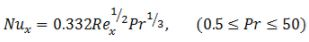(4.13)

where,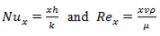and  are the local Nusselt and Reynold numbers, respectively.
An average value of the heat transfer coefficient over a distance l may be obtained by,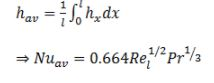(4.14)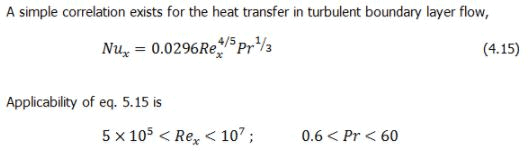Offer running on EduRev: Apply code STAYHOME200 to get INR 200 off on our premium plan EduRev Infinity!

## Heat Transfer for Engg.

46 videos|60 docs|59 tests

,

,

,

,

,

,

,

,

,

,

,

,

,

,

,

,

,

,

,

,

,

;